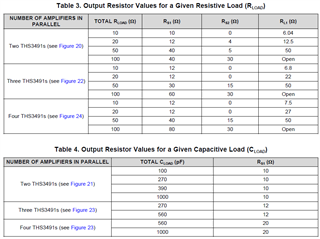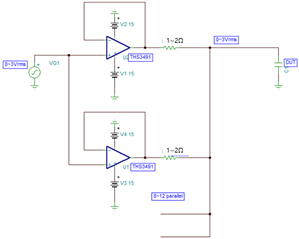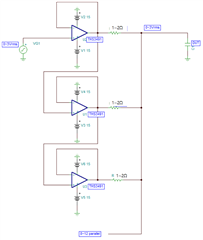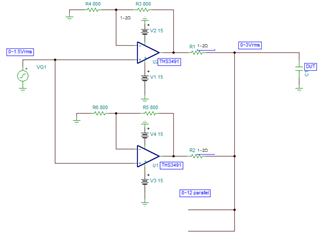If you have a related question, please click the "Ask a related question" button in the top right corner. The newly created question will be automatically linked to this question.

# THS3491: How much can reduce the Rs when parallelling as a power source

Part Number: THS3491

Hi Experts.

and I'm getting very valuable informations on this site and your advices.

This question is same project of my last thread.

I also have to make voltage source (buffer) to apply sinewave to DUT.

The Target is 3Apeak, and the frequency is 20~1MHz same as last thread.

So I'm try to use 8~12ea THS3491 like below. which is described on one of your document.But I can't use big Rs, I want to reduce it as possible as I can.

because if Rs is too big than DUT, Most of the voltage would be applied to Rs. not DUT.

So If possible, the equivalent Rs of all parallel Amps should be smaller than 100mohm.

Being such, the Rs of each amp should be 1.2ohm or smaller than that.

Could you give me a advice it would be possible or not?

Thank you

• You might look at this reference design,

https://www.ti.com/tool/TIDA-060002

• Hello Michael Steffes.

Thank you for sharing the document.

It is an honor to interest my thread.

Yes, Actually I don't fully understand yet but,

I think one of the important thing of this design guide is,

The differences of each output offset voltage would make some circulation current and

I have to consider the effect(power loss) when I design the circuit.

and there is also test parameters of Rs and Rg of it like below,but I think It's just test parameter. not recommended parameter or minimum value of it.

The reason why I ask you is,

10~20ohm which is described in that table is too big in my application.

and when the number of parallel and capacitance (Capacitive load) is more, the Rs value is bigger.

I have to use 10 or more Amps parallel,

So I'm worrying the Rs value should be more higher than that.

But it was too hard to find such answers in this design guide for me.

so I would like ask you it can be possible to reduce it 1~2ohm.

"Possible" means the stability is ok or not and malfunction would be appear some day.

So the point I'd like to ask you is, is It ok to use Rs as a 1ohm and

If possible, The only thing I have to consider is Power loss due to the offset voltage of each amp?

thank you again for your concerns.

• Hi Wonwoo,

what voltage signal amplitude do you need across the impedance "Z" you want to measure? This is essential for the dimensioning of circuit.

Kai

• Hi Kai,

Can the THS3491 operate as a voltage follower like below?or like below.If not, The circuit below also no problem. (I will use same value of Rg and Rf)Most important thing is, How much can the Rs could be minimized.

Because if equivalent Rs is too big, I have to apply big output voltage to keep the DUT voltage constant.

(If Rs = 1Ω and Z of DUT = 1Ω, If I have to give DUT 1V, The output voltage of THS3491 should be 1.414Volt.

but If Rs = 10Ω and Z of DUT = 1Ω , to give DUT 1V which is same as above, THS3491 have to support 10.05Volt.)

Thank you!

• Hi Wonwoo,

I would run the THS3491 at a gain of 5V/V:wonwoo_ths3491.TSC

And in order to reduce the heat dissipation of OPAmp, I would run the OPAmp at the lowest possible supply voltage. Using more OPAmps in parallel would also decrease the heat dissipation per OPAmp.

I would not recommend this paralleling scheme because of potential stability issues. So please run this circuit on your own risk. The higher the output resistors the lower the risk of instability...

Kai